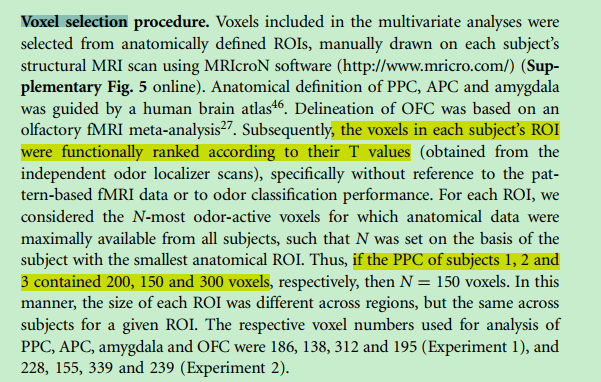# Voxel selectionHi all, how to functionally ranked the voxel according to their T values.

You could use Python code as below. You can also apply masks to only consider voxels inside a specific region. Below I apply a very simple mask: excluding voxels less than or equal to zero. However, this masking step could just as well refer to a region of interest image (where the region of interest had positive values).

``````import os
import numpy as np
import nibabel as nib

fnm = 'spmMotor.nii.gz'
data = img.get_fdata()
print("Intensity range: %g..%g"% (np.amin(data), np.amax(data)))
print("1st percentile: %g"% (np.percentile(data, 1)))
print("99th percentile: %g"% (np.percentile(data, 99)))
datapos = data[data>0]
print("1st percentile of positive values: %g"% (np.percentile(datapos, 1)))
print("99th percentile of positive values: %g"% (np.percentile(datapos, 99)))
``````

If you use the sample spm included with MRIcroGL you will get:

``````Intensity range: -6.86236..12.1565
1st percentile: -1.58045
99th percentile: 2.97615
1st percentile of positive values: 0.0211246
99th percentile of positive values: 5.81193``````

Thanks for you repaly.